NEET  >  31 Years NEET Previous Year Questions: Current Electricity - 2

# 31 Years NEET Previous Year Questions: Current Electricity - 2

Test Description

## 30 Questions MCQ Test Physics Class 12 | 31 Years NEET Previous Year Questions: Current Electricity - 2

31 Years NEET Previous Year Questions: Current Electricity - 2 for NEET 2023 is part of Physics Class 12 preparation. The 31 Years NEET Previous Year Questions: Current Electricity - 2 questions and answers have been prepared according to the NEET exam syllabus.The 31 Years NEET Previous Year Questions: Current Electricity - 2 MCQs are made for NEET 2023 Exam. Find important definitions, questions, notes, meanings, examples, exercises, MCQs and online tests for 31 Years NEET Previous Year Questions: Current Electricity - 2 below.
Solutions of 31 Years NEET Previous Year Questions: Current Electricity - 2 questions in English are available as part of our Physics Class 12 for NEET & 31 Years NEET Previous Year Questions: Current Electricity - 2 solutions in Hindi for Physics Class 12 course. Download more important topics, notes, lectures and mock test series for NEET Exam by signing up for free. Attempt 31 Years NEET Previous Year Questions: Current Electricity - 2 | 30 questions in 60 minutes | Mock test for NEET preparation | Free important questions MCQ to study Physics Class 12 for NEET Exam | Download free PDF with solutions
 1 Crore+ students have signed up on EduRev. Have you?
31 Years NEET Previous Year Questions: Current Electricity - 2 - Question 1

### In a metre-bridge, the balancing length from theleft end when standard resistance of 1 Ω is inright gap is found to be 20 cm. The value ofunknown resistance is 

Detailed Solution for 31 Years NEET Previous Year Questions: Current Electricity - 2 - Question 1

Let unknown resistance be X. Then
condition of Wheatstone's bridge gives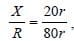where r is resistance of wire
per cm.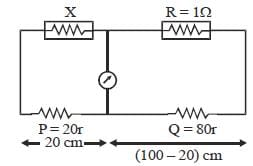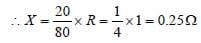31 Years NEET Previous Year Questions: Current Electricity - 2 - Question 2

### Two electric bulbs, one of 200 V, 40W and otherof 200 V, 100W are connected in a domesticcircuit. Then 

Detailed Solution for 31 Years NEET Previous Year Questions: Current Electricity - 2 - Question 2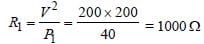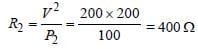∴ R1 (for 40W) > R2 (for 100 W)

31 Years NEET Previous Year Questions: Current Electricity - 2 - Question 3

### In electrolysis, the amount of mass deposited orliberated at an electrode is directly proportionalto 

Detailed Solution for 31 Years NEET Previous Year Questions: Current Electricity - 2 - Question 3

Amount deposited (m) = Zit = Zq
m ∝ q
Amount deposited is directly proportional
to charge.

31 Years NEET Previous Year Questions: Current Electricity - 2 - Question 4

Potentiometer measures potential moreaccurately because 

Detailed Solution for 31 Years NEET Previous Year Questions: Current Electricity - 2 - Question 4

Potentiometer measures potential current
more accurately because it measure potential
in open circuit and hence error in potential
due to internal resistance is removed.

31 Years NEET Previous Year Questions: Current Electricity - 2 - Question 5

Five resistances have been connected as shown in the figure. The effective resistance between A and B is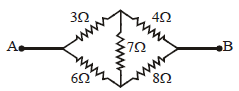Detailed Solution for 31 Years NEET Previous Year Questions: Current Electricity - 2 - Question 5

It is a balanced wheatstone bridge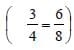, so the 7Ω resistance is
ineffective.
Equivalent resistance of 3Ω and 4Ω = 3 + 4
= 7Ω (series)
Equivalent resistance of 6Ω and 8Ω = 6 + 8
=14Ω (series)
Equivalent resistance of 7Ω and 14Ω (parallel)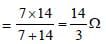31 Years NEET Previous Year Questions: Current Electricity - 2 - Question 6

A car battery has e.m.f. 12 volt and internalresistance 5 × 10–2 ohm. If it draws 60 amp current,the terminal voltage of the battery will be   

Detailed Solution for 31 Years NEET Previous Year Questions: Current Electricity - 2 - Question 6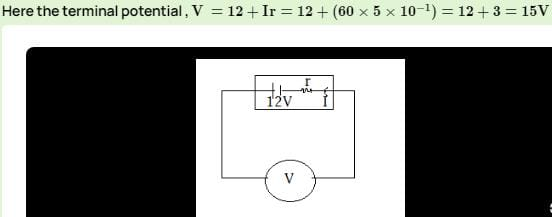31 Years NEET Previous Year Questions: Current Electricity - 2 - Question 7

Si and Cu are cooled to a temperature of 300 K,then resistivity? 

Detailed Solution for 31 Years NEET Previous Year Questions: Current Electricity - 2 - Question 7

For metals specific resistance decrease with decrease in temperature where as for semiconductors specific resistance increases with decrease in temperature.

31 Years NEET Previous Year Questions: Current Electricity - 2 - Question 8

If 25W, 220 V and 100 W, 220 V bulbs areconnected in series across a 440 V line, then    

Detailed Solution for 31 Years NEET Previous Year Questions: Current Electricity - 2 - Question 8

As for an electric appliance R = (Vs2 /W) ,
so for same specified voltage Vs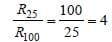i.e, R25 = 4R with R100 = R
Now in series potential divides in proportion
to resistance.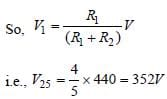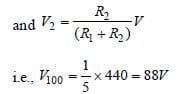From this, it is clear that voltage across 100
W bulb (= 88 V) is lesser than specified
(220 V) while across 25 W bulb (= 352 V) is
greater than specified (220 V), so, 25 W bulb
will fuse.

31 Years NEET Previous Year Questions: Current Electricity - 2 - Question 9

A battery of 10 V and internal resistance 0.5Ω isconnected across a variable resistance R. Thevalue of R for which the power delivered ismaximum is equal to [1992, 2001]

Detailed Solution for 31 Years NEET Previous Year Questions: Current Electricity - 2 - Question 9

Power is maximum when r = R, R = r = 0.5Ω.

31 Years NEET Previous Year Questions: Current Electricity - 2 - Question 10

A wire has a resistance of 3.1Ω at 30ºC and aresistance 4.5Ω at 100ºC. The temperaturecoefficient of resistance of the wire 

Detailed Solution for 31 Years NEET Previous Year Questions: Current Electricity - 2 - Question 10

R1 = 3.1 Ω at t = 30°C
R2 = 4.5 Ω at t = 100°C
We have, R = R0 (1 + αt)
∴ R1 = R0 [1 + α (30)]
R2 = R0 [1 + α (100)]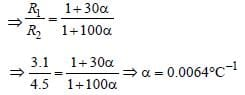31 Years NEET Previous Year Questions: Current Electricity - 2 - Question 11

If specific resistance of a potentiometer wire is10–7 Ωm, the current flow through it is 0.1 A andthe cross-sectional area of wire is 10–6 m2 thenpotential gradient will be 

Detailed Solution for 31 Years NEET Previous Year Questions: Current Electricity - 2 - Question 11

Potential gradient = Potential fall per unit
length. In this case resistance of unit length.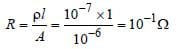Potential fall across R is

V = I.R = 0.1x10-1 = 0.01 volt/m.
= 10-2 volt /m

31 Years NEET Previous Year Questions: Current Electricity - 2 - Question 12

The resistivity (specific resistance) of a copperwire 

Detailed Solution for 31 Years NEET Previous Year Questions: Current Electricity - 2 - Question 12

Resistivity of copper wire increases with
increase in temprature as ρt = ρ0 (1+ αt)
Copper being a metal has positive coefficient
of resistivity.

31 Years NEET Previous Year Questions: Current Electricity - 2 - Question 13

The potential difference between the terminals of a cell in an open circuit is 2.2 V. When a resistor of 5Ω is connected across the terminals of the cell, the potential difference between the terminals of the cell is found to be 1.8 V. The internal resistance of the cell is 

Detailed Solution for 31 Years NEET Previous Year Questions: Current Electricity - 2 - Question 13

E = V + ir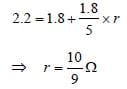31 Years NEET Previous Year Questions: Current Electricity - 2 - Question 14

An electric kettle has two heating coils. Whenone of the coils is connected to an a.c. source,the water in the kettle boils in 10 minutes. Whenthe other coil is used, the water boils in 40minutes. If both the coils are connected inparallel, the time taken by the same quantity ofwater to boil will be    

Detailed Solution for 31 Years NEET Previous Year Questions: Current Electricity - 2 - Question 14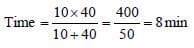31 Years NEET Previous Year Questions: Current Electricity - 2 - Question 15

Two 220 volt, 100 watt bulbs are connected firstin series and then in parallel. Each time thecombination is connected to a 220 volt a.c.supply line. The power drawn by thecombination in each case respectively will be   

Detailed Solution for 31 Years NEET Previous Year Questions: Current Electricity - 2 - Question 15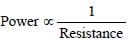In series combination, resistance doubles.

Hence, power will be halved.
In parallel combination, resistance halves.
Hence, power will be doubled.

31 Years NEET Previous Year Questions: Current Electricity - 2 - Question 16

Fuse wire is a wire of    

Detailed Solution for 31 Years NEET Previous Year Questions: Current Electricity - 2 - Question 16

Fuse wire : It is used in a circuit to control the
maximum current flowing in circuit. It is a thin
wire having high resistance and is made up
of a material with low melting point.

31 Years NEET Previous Year Questions: Current Electricity - 2 - Question 17

In a Wheatstone’s bridge all the four arms have equal resistance R. If the resistance of the galvanometer arm is also R, the equivalent resistance of the combination as seen by the battery is    

Detailed Solution for 31 Years NEET Previous Year Questions: Current Electricity - 2 - Question 17

Since, Wheatstone's bridge is balanced, then
resistance of galvanometer will be
uneffective.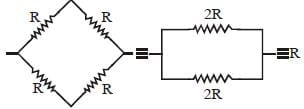31 Years NEET Previous Year Questions: Current Electricity - 2 - Question 18

A battery is charged at a potential of 15V for 8hours when the current flowing is 10A. Thebattery on discharge supplies a current of 5Afor 15 hours. The mean terminal voltage duringdischarge is 14V. The “watt-hour” efficiency ofthe battery is 

Detailed Solution for 31 Years NEET Previous Year Questions: Current Electricity - 2 - Question 18

Efficiency is given by η = output/input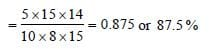31 Years NEET Previous Year Questions: Current Electricity - 2 - Question 19

When three identical bulbs of 60 watt, 200 voltrating are connected in series to a 200 voltsupply, the power drawn by them will be        

Detailed Solution for 31 Years NEET Previous Year Questions: Current Electricity - 2 - Question 19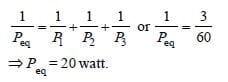31 Years NEET Previous Year Questions: Current Electricity - 2 - Question 20

In India electricity is supplied for domestic useat 220 V. It is supplied at 110 V in USA. If theresistance of a 60 W bulb for use in India is R,the resistance of a 60 W bulb for use in USA will  be    

Detailed Solution for 31 Years NEET Previous Year Questions: Current Electricity - 2 - Question 20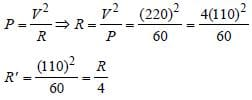31 Years NEET Previous Year Questions: Current Electricity - 2 - Question 21

The electric resistance of a certain wire of iron isR. If its length and radius are both doubled, then

Detailed Solution for 31 Years NEET Previous Year Questions: Current Electricity - 2 - Question 21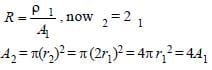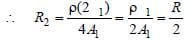∴ Resistance is halved, but specific
resistance remains the same.

31 Years NEET Previous Year Questions: Current Electricity - 2 - Question 22

Resistances n, each of r ohm, when connectedin parallel give an equivalent resistance of Rohm. If these resistances were connected inseries, the combination would have a resistancein ohms, equal to 

Detailed Solution for 31 Years NEET Previous Year Questions: Current Electricity - 2 - Question 22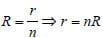When connected in series, Req = nr
= n (nR) = n2R

31 Years NEET Previous Year Questions: Current Electricity - 2 - Question 23

Five equal resistances each of resistance R are connected as shown in the figure. A battery of V volts is connected between A and B. The current flowing in AFCEB will be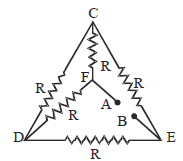Detailed Solution for 31 Years NEET Previous Year Questions: Current Electricity - 2 - Question 23

A balanced Wheststone’s bridge exists
between A & B.

∴ Req = R
Current through circuit = V/R
Current through AFCEB = V/2R

31 Years NEET Previous Year Questions: Current Electricity - 2 - Question 24

A 6 volt battery is connected to the terminals ofthe three metre long wire of uniform thicknessand resistance of 100 ohm. The difference ofpotential between two points on the wireseparated by a distance of 50 cm will be  

Detailed Solution for 31 Years NEET Previous Year Questions: Current Electricity - 2 - Question 24

R α
For 300 cm, R = 100 Ω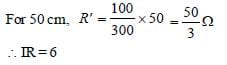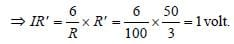31 Years NEET Previous Year Questions: Current Electricity - 2 - Question 25

A 5–ampere fuse wire can withstand amaximum power of 1 watt in the circuit. Theresistance of the fuse wire is 

Detailed Solution for 31 Years NEET Previous Year Questions: Current Electricity - 2 - Question 25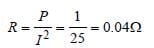31 Years NEET Previous Year Questions: Current Electricity - 2 - Question 26

When a wire of uniform cross–section a, length l and resistance R is bent into a complete circle, resistance between any two
of diametrically opposite points will be 

Detailed Solution for 31 Years NEET Previous Year Questions: Current Electricity - 2 - Question 26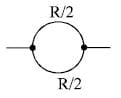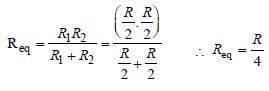31 Years NEET Previous Year Questions: Current Electricity - 2 - Question 27

For the network shown in the Fig. the value of the current i is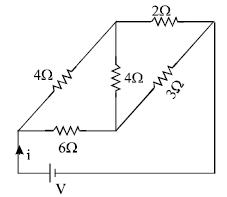Detailed Solution for 31 Years NEET Previous Year Questions: Current Electricity - 2 - Question 27

It is a balanced Wheatstone bridge. Hence
resistance 4Ω can be eliminated.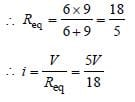31 Years NEET Previous Year Questions: Current Electricity - 2 - Question 28

Two batteries, one of emf 18 volt and internal resistance 2Ω and the other of emf 12 volt andinternal resistance 1Ω, are connected asshown. The voltmeter V will record a readingof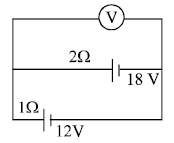Detailed Solution for 31 Years NEET Previous Year Questions: Current Electricity - 2 - Question 28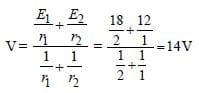(Since the cells are in parallel).

31 Years NEET Previous Year Questions: Current Electricity - 2 - Question 29

In producing chlorine through electrolysis, 100watt power at 125 V is being consumed. Howmuch chlorine per minute is liberated? E.C.E. ofchlorine is 0.367 × 10–6 kg/ coulomb. 

Detailed Solution for 31 Years NEET Previous Year Questions: Current Electricity - 2 - Question 29

Power = V × I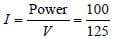E.C.E. of chlorine is 0.367 × 10–6 kg/coulomb
Charge passing in one minute =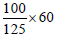= 48 coulomb

Chlorine precipitated = 0.367 × 10–6 × 48
= 17.6 × 10–6 kg
= 17.6 mg

31 Years NEET Previous Year Questions: Current Electricity - 2 - Question 30

Power dissipated across the 8Ω resistor in the circuit shown here is 2 watt. The power dissipated in watt units across the 3Ω resistor is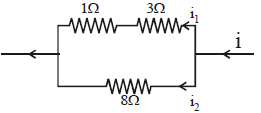Detailed Solution for 31 Years NEET Previous Year Questions: Current Electricity - 2 - Question 30

Power = V . I = I2R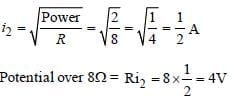This is the potential over parallel branch. So,

i1 = 4/4 =1A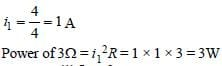## Physics Class 12

157 videos|452 docs|213 tests
 Use Code STAYHOME200 and get INR 200 additional OFF Use Coupon Code
Information about 31 Years NEET Previous Year Questions: Current Electricity - 2 Page
In this test you can find the Exam questions for 31 Years NEET Previous Year Questions: Current Electricity - 2 solved & explained in the simplest way possible. Besides giving Questions and answers for 31 Years NEET Previous Year Questions: Current Electricity - 2, EduRev gives you an ample number of Online tests for practice

## Physics Class 12

157 videos|452 docs|213 tests

### How to Prepare for NEET

Read our guide to prepare for NEET which is created by Toppers & the best Teachers